Author: Oscar Cronquist Article last updated on November 11, 2019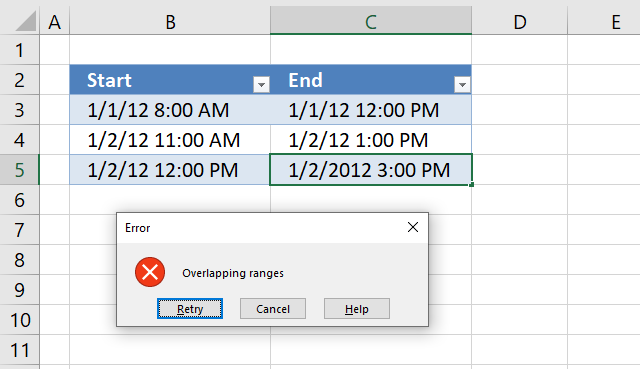The picture above shows an Excel Table with Data Validation applied. An error dialog box appears if a user tries to enter an overlapping date and time range.

The image above shows an error dialog box that showed up because the date range 1/2/12 12:00 PM - 1/2/12 3:00 PM overlaps 1/2/12 11:00 AM - 1/2/12 1:00 PM.

Both the start and end of the date range must be entered to trigger the error dialog box provided the ranges overlap.

Data Validation is a feature in Excel that allows you to check values entered in your worksheet, this webpage explains it in great detail:  What is Data Validation?

I am going to show you how to use a Data Validation formula in this article that checks date ranges and will warn the user if the date ranges overlap.

### Create Data Validation with a custom formula

If you apply Data Validation to an Excel defined Table, all new values you enter below the last value in the Excel Table will have the same Data Validation setting applied.

In other word, the Excel table copies the Data Validation automatically to new cells when the Excel Table grows which is great and saves you time.

1. Select all values except the headers in the Excel Table.
2. Go to tab "Data" on the ribbon.
3. Press with left mouse button on "Data Validation" button.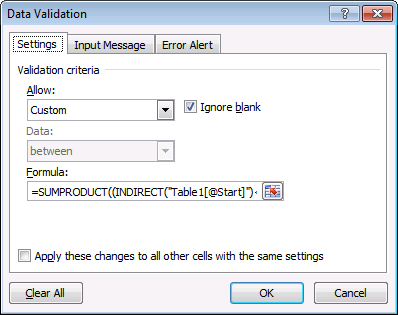4. Press with left mouse button on the drop-down list below "Allow:" and select "Custom", see image above.
5. Type or copy/paste the following formula:
=SUMPRODUCT((INDIRECT("Table1[@Start]")<=INDIRECT("Table1[End]"))* (INDIRECT("Table1[@End]")>=INDIRECT("Table1[Start]")))<=1
6. Press with left mouse button on OK button to apply Data Validation.

To be able to use Excel defined Tables in Data Validation formulas you have a few options which I have described in this article: How to use an Excel Table name in Data Validation Lists and Conditional Formatting formulas

One workaround demonstrated in the article is to use the INDIRECT function each time you reference an Excel Table, I will now explain the formula in great detail.

### Explaining Data Validation formula in cell C5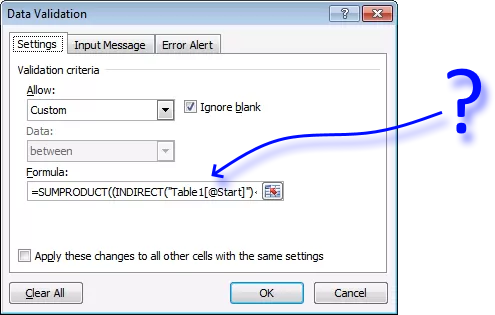A great feature with Excel Tables is that a reference pointing to an Excel Table doesn't change (unless you change the Excel Table name or headers) even if the Table grows or shrinks, the reference stays the same.

By the way, they are called structured references and they behave differently than regular cell references.

If you want to see each calculation step using the Evaluate Formula tool then copy the formula and paste to any empty cell not adjacent to the Excel Table, we will delete it later on.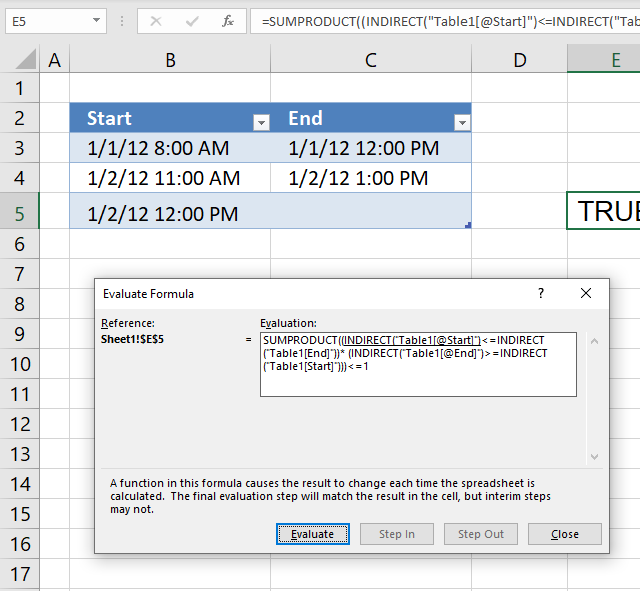Go to tab "Formula" on the ribbon and press with left mouse button on the "Evaluate Formula" button, this opens a dialog box that allows you to see each step in the calculation.

Simply press with left mouse button on the Evaluate button located on the dialog box to go to next step, keep press with left mouse button oning to see all calculation steps.

#### Step 1 - Check if start date is smaller than or equal to the end dates

Table1[@Start] is a reference to a cell on the same row as the selected cell and in column Start in Table1.

The less than sign and the equal sign combined checks if the value in the cell described above is less than or equal to the value in column End in Table1.

The less than and equal sign are logical operators and return a boolean value TRUE or FALSE. Table1[@Start] is a reference to a cell in column Start that is located on the same row as the current cell, in this case, cell C5. The @ character in Table1[@Start] means it is on the same row as the selected cell.

An Excel date is actually a number formatted as a date, 1/1/1900 is 1 and 1/1/2000 is 36526. There are 36525 days between 1/1/1900 and 1/1/2000.

Time is a fraction of a day, one hour is 1/24 and is approx. 0.041667 and 24 hours is 1. 12:00 PM is 0.5.

INDIRECT("Table1[@Start]")<=INDIRECT("Table1[End]")

becomes

"1/2/2012 12:00 PM"<={"1/1/12 12:00 PM",  "1/2/12 1:00 PM", "1/2/12 3:00 PM"}

becomes

40910.5<={40909.5; 40910.5416666667; 40910.625}

and returns {FALSE; TRUE; TRUE}.

#### Step 2 - Check if end date is larger than or equal to the start dates

INDIRECT("Table1[@End]")>=INDIRECT("Table1[Start]")

becomes

"1/2/2012">={"1/1/12 8:00 AM", "1/2/12 11:00 AM", "1/2/12 12:00 PM"}

becomes

40910.625>={40909.3333333333; 40910.4583333333; 40910.5}

and returns {TRUE; TRUE; TRUE}.

#### Step 3 - Apply AND logic and sum array

The parentheses let you control the order of calculations, the asterisk multiplies the arrays which means that we apply AND logic meaning both values on the same row must return TRUE in order to return TRUE.

When you multiply boolean values Excel converts the output to their numerical equivalents, TRUE = 1 and FALSE = 0 (zero).

SUMPRODUCT((INDIRECT("Table1[@Start]")<=INDIRECT("Table1[End]"))* (INDIRECT("Table1[@End]")>=INDIRECT("Table1[Start]")))

becomes

SUMPRODUCT({FALSE; TRUE; TRUE}*{TRUE; TRUE; TRUE})

becomes

SUMPRODUCT({0; 1; 1})

and returns 2.

#### Step 4 - Check if value is less or equal to 1

If the number returned from the SUMPRODUCT function is larger than 1 we know that there is an overlapping date range.

The formula compares all ranges so the range we entered is also calculated, this makes the formula always return 1 for that row. That is why we need to check if the value is greater than 1.

SUMPRODUCT((INDIRECT("Table1[@Start]")<=INDIRECT("Table1[End]"))* (INDIRECT("Table1[@End]")>=INDIRECT("Table1[Start]")))<=1

becomes

2<=1 and returns FALSE. The error dialog box is shown.

### Customize Data Validation Error Alert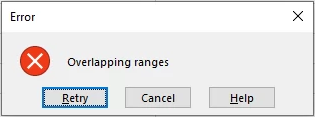1. Select any value in the Excel Table.
2. Go to tab "Data" on the ribbon.
3. Press with left mouse button on the "Data Validation" button and a dialog box appears.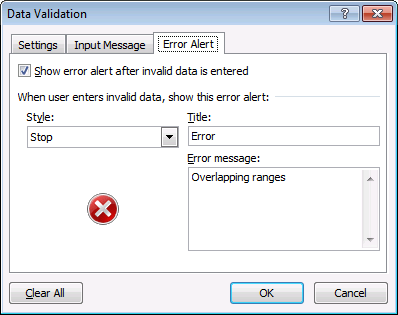4. Go to tab "Error Alert", shown in the image above.
5. Enter a title.
6. Enter error message. You can also choose a style.
7. Press with left mouse button on OK button.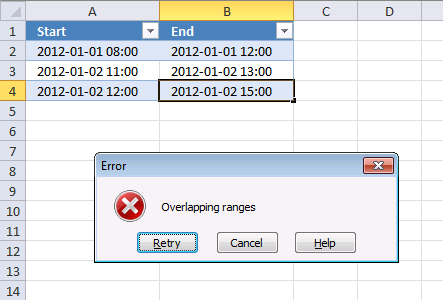### Get the Excel filePrevent-users-from-entering-overlapping-date-and-time-ranges.xlsx